# Class 9 RD Sharma Solutions – Chapter 10 Congruent Triangles- Exercise 10.6

• Last Updated : 28 Mar, 2021

### Question 1: In ΔABC, if ∠A = 40° and ∠B = 60°. Determine the longest and shortest sides of the triangle.

Solution: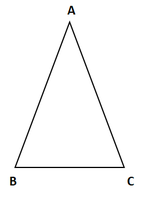Given that in ΔABC, ∠A = 40° and ∠B = 60°

To find: longest and shortest side

We know that,

Sum of angles in a triangle 180°

∠A + ∠B + ∠C = 180°

40° + 60° + ∠C = 180°

∠C = 180° – 100° = 80°

∠C = 80°

Now,

⟹ 40° < 60° < 80° = ∠A < ∠B < ∠C

⟹ ∠C is greater angle and ∠A is smaller angle.

Now, ∠A < ∠B < ∠C

⟹ BC < AC < AB [Side opposite to greater angle is larger and side opposite to smaller angle is smaller]

AB is longest and BC is the shortest side.

### Question 2: In a ΔABC, if ∠B = ∠C = 45°, which is the longest side?

Solution: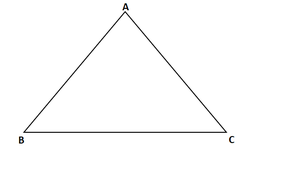Given that in ΔABC,

ΔABC, ∠B = ∠C = 45°

To find: longest side

We know that.

Sum of angles in a triangle =180°

∠A + ∠B + ∠C = 180°

∠A + 45° + 45° = 180°

∠A = 180° – (45° + 45°) = 180° – 90° = 90°

∠A = 90°

### Question 3: In ΔABC, side AB is produced to D so that BD = BC. If ∠B = 60° and ∠A = 70°. Prove that: (i) AD > CD (ii) AD > AC

Solution: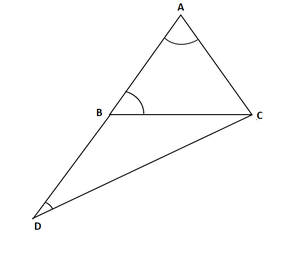Given that, in Δ ABC, side AB is produced to D so that BD = BC.

∠B = 60°, and ∠A = 70°

To prove:

First join C and D

We know that,

Sum of angles in a triangle =180°

∠A + ∠B + ∠C = 180°

70° + 60° + ∠C = 180°

∠C = 180° – (130°) = 50°

∠C = 50°

∠ACB = 50° … (i)

Also in Δ BDC

∠DBC =180 – ∠ABC [ABD is a straight angle]

180 – 60° = 120°

and also BD = BC[given]

∠BCD = ∠BDC [Angles opposite to equal sides are equal]

Now,

∠DBC + ∠BCD + ∠BDC = 180° [Sum of angles in a triangle =180°]

⟹ 120° + ∠BCD + ∠BCD = 180°

⟹ 120° + 2∠ BCD = 180°

⟹ 2∠BCD = 180° – 120° = 60°

⟹ ∠BCD = 30°

⟹ ∠BCD = ∠BDC = 30° …. (ii)

∠BAC ⟹ ∠DAC = 70° [given]

∠BDC ⟹ ∠ADC = 30° [From (ii)]

∠ACD = ∠ACB + ∠BCD

= 50° + 30°[From (i) and (ii)] = 80°

Now, ∠ADC < ∠DAC < ∠ACD

AC < DC < AD [Side opposite to greater angle is longer and smaller angle is smaller]

Hence proved

### Question: 4 Is it possible to draw a triangle with sides of length 2 cm, 3 cm, and 7 cm?

Solution:

Given lengths of sides are 2cm, 3cm and 7cm.

To check whether it is possible to draw a triangle with the given lengths of sides

We know that,

A triangle can be drawn only when the sum of any two sides is greater than the third side.

So, let’s check the rule.

2 + 3 < 7 [the sum of any two sides is not greater than the third side, so not satisfying the triangle condition]

2 + 7 > 3

and 3 + 7 > 2

So, the triangle does not exit.

### Question: 5 O is any point in the interior of ΔABC. Prove that.

(i) AB + AC > OB + OC

(ii) AB + BC + CA > OA + QB + OC

(iii) OA + OB + OC > (1/2)(AB + BC +CA)

Solution: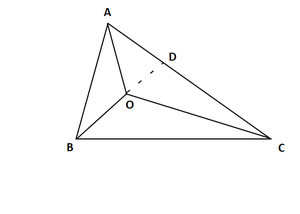Given that O is any point in the interior of ΔABC

To prove

(i) AB + AC > OB + OC

(ii) AB + BC + CA > OA + QB + OC

(iii) OA + OB + OC > (1/2)(AB + BC +CA)

We know that in a triangle the sum of any two sides is greater than the third side.

So, we have

In Δ ABC

AB + BC > AC

BC + AC > AB

AC + AB > BC

In ΔOBC

OB + OC > BC … (i)

In ΔOAC

OA + OC > AC … (ii)

In ΔOAB

OA + OB > AB … (iii)

Now, extend BO to meet AC in D.

Now, in ΔABD, we have

AB + AD > BO + OD … (iv)  [BD = BO + OD]

Similarly, in ΔODC, we have

OD + DC > OC … (v)

(i) Adding (iv) and (v), we get

AB + AD + OD + DC > BO + OD + OC

AB + (AD + DC) > OB + OC

AB + AC > OB + OC … (vi)

Similarly, we have

BC + BA > OA + OC … (vii)

and CA+ CB > OA + OB … (viii)

(ii) Adding equation (vi), (vii) and (viii), we get

AB + AC + BC + BA + CA + CB > OB + OC + OA + OC + OA + OB

⟹ 2AB + 2BC + 2CA > 2OA + 2OB + 2OC

⟹ 2(AB + BC + CA) > 2(OA + OB + OC)

⟹ AB + BC + CA > OA + OB + OC

(iii) Adding equations (i), (ii) and (iii)

OB + OC + OA + OC + OA + OB > BC + AC + AB

2OA + 2OB + 2OC > AB + BC + CA

We get = 2(OA + OB + OC) > AB + BC +CA

(OA + OB + OC) > (1/2)(AB + BC +CA)

### Question: 6 Prove that the perimeter of a triangle is greater than the sum of its altitudes.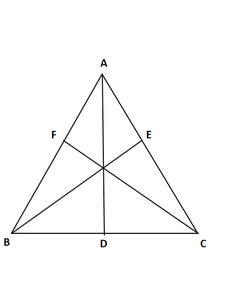Proof:

We know that of all the segments that can be drawn to a given line, from a point not lying on it, the perpendicular distance i.e, the perpendicular line segment is the shortest.

Therefore

AB + AC > 2AD …. (i)

BE ⊥ AC

BA > BE and BC > BE

BA + BC > 2BE … (ii)

CF ⊥ AB

CA > CF and CB > CF

CA + CB > 2CF … (iii)

Adding (i), (ii) and (iii), we get

AB + AC + BA + BC + CA + CB > 2AD + 2BE + 2CF

2AB + 2BC + 2CA > 2(AD + BE + CF)

AB + BC + CA > AD + BE + CF

The perimeter of the triangle is greater than that the sum of its altitudes

Hence proved

Question 7: In Fig., prove that:

(i) CD + DA + AB + BC > 2AC

(ii) CD + DA + AB > BC

Solution: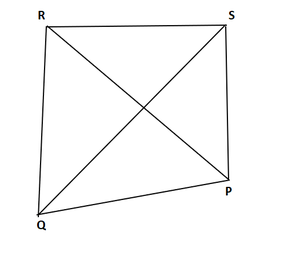To prove

(i) CD + DA + AB + BC > 2AC

(ii) CD + DA+ AB > BC

We know that, in a triangle sum of any two sides is greater than the third side

(i) So,

In ΔABC, we have

AB + BC > AC  …. (i)

CD + DA > AC …. (ii)

Adding (i) and (ii), we get

AB + BC + CD + DA > AC + AC

AB + BC + CD + DA > 2AC

(ii) Now, In Δ ABC, we have,

AB + AC > BC … (iii)

CD + DA > AC

CD + DA + AB > AC + AB

From equation (iii) and (iv), we get,

CD + DA + AB > AC + AB > BC

CD + DA + AB > BC

Hence proved

### Question 8: Which of the following statements are true (T) and which are false (F)?

(i) Sum of the three sides of a triangle is less than the sum of its three altitudes.

(ii) Sum of any two sides of a triangle is greater than twice the median drown to the third side

(iii) Sum of any two sides of a triangle is greater than the third side.

(iv) Difference of any two sides of a triangle is equal to the third side.

(v) If two angles of a triangle are unequal, then the greater angle has the larger side opposite to it

(vi) Of all the line segments that can be drawn from a point to a line not containing it, the perpendicular line segment is the shortest one.

Solution:

(i) False because sum of three sides of a triangle is greater than sum of its three altitudes

(ii) True

(iii) True

(iv) False because the difference of any two sides of a triangle is less than third side.

(v) True because the side opposite to the greatest angle is longest in a triangle.

(vi) True because the perpendicular distance is the shortest distance from a point to a line not containing it.

### Question 9: Fill in the blanks to make the following statements true.

(i) In a right triangle the hypotenuse is the ____________ side.

(ii) The sum of three altitudes of a triangle is ____________ than its perimeter.

(iii) The sum of any two sides of a triangle is ____________ than the third side.

(iv) If two angles of a triangle are unequal, then the smaller angle has the ____________ side opposite to it.

(v) Difference of any two sides of a triangle is ____________ than the third side.

(vi) If two sides of a triangle are unequal, then the larger side has ____________ angle opposite to it.

Solution:

(i) In a right triangle the hypotenuse is the largest side

(ii) The sum of three altitudes of a triangle is less than its perimeter.

(iii) The sum of any two sides of a triangle is greater than the third side.

(iv) If two angles of a triangle are unequal, then the smaller angle has the smaller side opposite to it.

(v) Difference of any two sides of a triangle is less than the third side.

(vi) If two sides of a triangle are unequal, then the larger side has greater angle opposite to it.

My Personal Notes arrow_drop_up